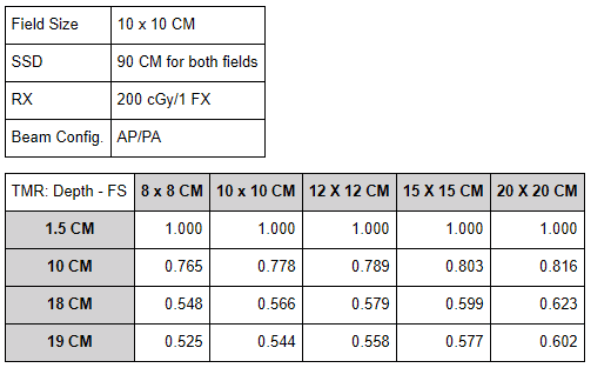The following equations are used for TMR calculations:

1. TMR = dose at depth in tissue for field size (D_dx) / dose at depth of maximum dose for the same field size (D_dmax).
2. Dose at depth = dose at isocenter * (TMR_depth in tissue / TMR_depth of isocenter) * inverse square correction.
3. Equivalent Square = 4 * Area (A) / Perimeter (P).
4. Inverse square correction for shallower depth relative to isocenter = (farther point distance / closer point distance) ^2.
5. Inverse square correction for deeper depth relative to isocenter = (closer point distance / farther point distance) ^ 2.
• For the last two equations, the arrangement of the distances (numerator/denominator) will depend on whether you are calculating for a point distance shallower to or deeper to the isocenter. Through any medium radiation passes through, the greater depth a beam traverses, the more the beam will depreciate by attenuation. Calculating for a point distance deeper to the isocenter will result in a lesser absorbed dose relative to a shallower point. On the contrary, calculating for a point distance shallower to the isocenter will result in a greater absorbed dose relative to a deeper point.• Find the dose at 1.5 CM depth from the AP direction

The following are the calculations for the AP beam:

1. Calculate for projected field size at distance of 91.5 CM: (10/100)*91.5 = 9.15 X 9.15 CM
2. Interpolate for TMR at 1.5 cm depth w/ projected field size of 9.15 CM: {[(1.000-1.000)/20]*1.5}+1.000 = 1.000
3. Look up TMR at 10 CM depth w/ field size of 10 CM: 0.778
4. Calculate for dose at 1.5 CM depth before inverse square law correction: 100*(1.000/0.788) = 128.53 cGy
5. Apply inverse square law correction for dose at 1.5 CM depth: 128.53*(100/91.5)^2 = 153.52 cGy

The following are the calculations for the PA beam:

1. Calculate for the projected field size at distance of 108.5 CM: (10/100)*108.5 = 10.85 X 10.85 CM
2. Interpolate for TMR at 18 CM depth w/ projected field size of 10.85 CM: {[(0.579-0.566)/20]*8.5}+0.566 = 0.572
3. Interpolate for TMR at 19 CM depth w/ projected field size of 10.85 CM: {[(0.558-0.544)/20]*8.5}+0.544 = 0.550
4. Interpolate for TMR at 18.5 CM depth w/ projected field size of 10.85 CM: [(0.550-0.572)/2]+0.572 = 0.561
5. Calculate for dose at 18.5 CM depth before inverse square law correction: 100*(0.561/0.778) = 72.11 cGy
6. Apply inverse square law correction for dose at 18.5 CM depth: 72.11*(100/108.5)^2 = 61.25 cGy
7. Find total dose delivered: 153.52+61.25 = 214.77 cGy• Find the dose to the node at 5 CM off axis and 3 CM depth
1. Calculate for projected field size at distance of 93 CM: (15/100)*93 = 13.95 X 13.95 CM
2. Interpolate for TMR at 3 CM depth w/ projected field size of 13.95 CM: {[(0.980-0.980)/30]*19.5}+0.980 = 0.980
3. Look up TMR at 10 CM depth w/ field size of 15 CM: 0.803
4. Calculate for dose at 3 CM depth before inverse square law correction: 200*(0.980/0.803) = 244.08 cGy
5. Apply inverse square law correction for dose at 3 CM depth: 244.08*(100/93)^2 = 282.21 cGy
6. Interpolate for OAR at 5 CM off axis 3 CM depth: {[(1.026-1.031)/3.4]*1.37}+1.031 = 1.029
7. Apply OAR correction to find dose to node at 5 CM off axis and 3 CM depth: 282.21*1.029 = 290.39 cGy• Find the dose to a node at 5 CM off axis and 4 CM depth from the surface with a SSD of 91 CM
1. Calculate for projected field size at distance of 95 CM: (15/100)*95 = 14.25 X 14.25 CM
2. Interpolate for TMR at 4 CM depth w/ projected field size of 14.25 CM: {[(0.959-0.955)/30]*22.5}+0.955 = 0.958
3. Look up TMR at 10 CM depth w/ field size of 15 CM: 0.803
4. Calculate for dose at 4 CM depth before inverse square law correction: 200*(0.958/0.803) = 238.61 cGy
5. Apply inverse square law correction for dose at 4 CM depth: 238.61*(100/95)^2 = 264.39 cGy
6. Interpolate for OAR at 5 CM off axis 4 CM depth: {[(1.026-1.031)/3.4]*2.37}+1.026 = 1.028
7. Apply OAR correction to find dose to node at 5 CM off axis and 3 CM depth: 264.39*1.028 = 271.79 cGy